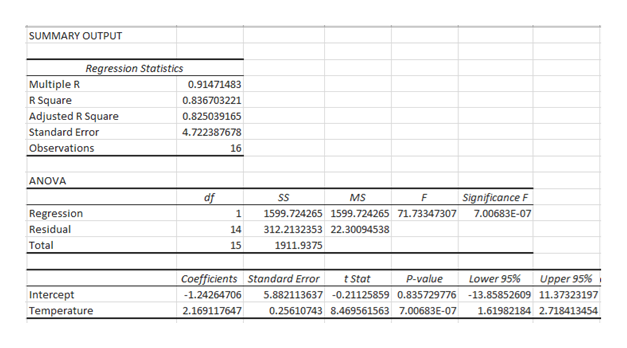# The toco toucan, the largest member of the toucan family, possesses the largest beak relative to body size of all birds. This exaggerated feature has received various interpretations, such as being a refined adaptation for feeding. However, the large surface area may also be an important mechanism for radiating heat (and hence cooling the bird) as outdoor temperature increases. Here are data for beak heat loss, as a percent of total body heat loss from all sources, at various temperatures in degrees Celsius. [Note: The numerical values in this problem have been modified for testing purposes.]Temperature (oC)(oC)15161718192021222324252627282930Percent heat loss from beak33333331374456524554465559596163The equation of the least-squares regression line for predicting beak heat loss, as a percent of total body heat loss from all sources, from temperature is: (Use decimal notation. Enter the values of the intercept and slope rounded to two decimal places. Use the letter x to represent the value of the temperature.) ?̂ =  Use the equation of the least‑squares regression line to predict beak heat loss, as a percent of total body heat loss from all sources, at a temperature of 25 degrees Celsius. Enter your answer rounded to two decimal places.beak heat as a percent of total body heat loss=beak heat as a percent of total body heat loss=% What percent of the variation in beak heat loss is explained by the straight-line relationship with temperature? Enter your answer rounded to two decimal places.percent of variation in beak heat loss explained by the equation=percent of variation in beak heat loss explained by the equation= %Find the correlation r between beak heat loss and temperature. Enter your answer rounded to three decimal places.?=

Question
1433 views
The toco toucan, the largest member of the toucan family, possesses the largest beak relative to body size of all birds. This exaggerated feature has received various interpretations, such as being a refined adaptation for feeding. However, the large surface area may also be an important mechanism for radiating heat (and hence cooling the bird) as outdoor temperature increases. Here are data for beak heat loss, as a percent of total body heat loss from all sources, at various temperatures in degrees Celsius. [Note: The numerical values in this problem have been modified for testing purposes.]
 Temperature (oC)(oC) Percent heat loss from beak 15 16 17 18 19 20 21 22 23 24 25 26 27 28 29 30 33 33 33 31 37 44 56 52 45 54 46 55 59 59 61 63
The equation of the least-squares regression line for predicting beak heat loss, as a percent of total body heat loss from all sources, from temperature is: (Use decimal notation. Enter the values of the intercept and slope rounded to two decimal places. Use the letter x to represent the value of the temperature.)

?̂ =

Use the equation of the least‑squares regression line to predict beak heat loss, as a percent of total body heat loss from all sources, at a temperature of 25 degrees Celsius. Enter your answer rounded to two decimal places.
beak heat as a percent of total body heat loss=beak heat as a percent of total body heat loss=
%

What percent of the variation in beak heat loss is explained by the straight-line relationship with temperature? Enter your answer rounded to two decimal places.
percent of variation in beak heat loss explained by the equation=percent of variation in beak heat loss explained by the equation=

%
Find the correlation r between beak heat loss and temperature. Enter your answer rounded to three decimal places.
?=
check_circle

Step 1

The regression analysis is conducted here by using EXCEL. The software procedure is given below:

• Enter the data as Temperature in column A and percent heat loss from break in column B.
• Select Data > Data Analysis > Regression> OK.
• Enter Input Y Range as \$B\$1:\$B\$17.
• Enter Input X Range as \$A\$1:\$A\$17.
• Click Labels.
• Click OK.

The output using EXCEL is as follows:help_outlineImage TranscriptioncloseSUMMARY OUTPUT Regression Statistics Multiple R 0.91471483 R Square 0.836703221 Adjusted R Square 0.825039165 Standard Error 4.722387678 Observations 16 ANOVA Significance F df SS MS Regression 1 1599.724265 1599.724265 71.73347307 7.00683E-07 Residual 14 312.2132353 22.30094538 Total 15 1911.9375 Upper 95% Coefficients Standard Error P-value Lower 95% tStat Intercept -1.24264706 5.882113637 -0.21125859 0.835729776 -13.85852609 11.37323197 Temperature 2.169117647 0.25610743 8.469561563 7.00683E-07 1.61982184 2.718413454 fullscreen
Step 2

Let y be the expected value of temperature at given value of percent heat loss from break.

Then the least squares regression equation for predicting break heat loss is given by,

y = –1.24265 + 2.16912 (T...

### Want to see the full answer?

See Solution

#### Want to see this answer and more?

Solutions are written by subject experts who are available 24/7. Questions are typically answered within 1 hour.*

See Solution
*Response times may vary by subject and question.
Tagged in
MathStatistics